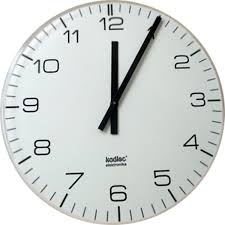# Three people

Three people start doing a work at a same time. The first worked only 2 hours. The second ended 3 hours before the end. On an individual basis, it would take the first time to do the work 10 hours, second 12 hours and 15 hours third. How many hours did it take to work together under these conditions?

t =  7 h

### Step-by-step explanation:

2/10 + (t-3)/12 + t/15 = 1

9t = 63

t = 63/9 = 7

t = 7

Our simple equation calculator calculates it.Did you find an error or inaccuracy? Feel free to write us. Thank you!

Tips for related online calculators
Do you have a linear equation or system of equations and looking for its solution? Or do you have a quadratic equation?
Do you want to convert time units like minutes to seconds?